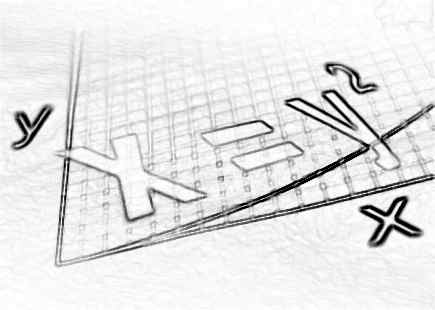Mar
19
2011

# Who Invented Algebra

Algebra is the major branch of mathematics that deals with study of operations and relation, construction methods, concepts, polynomial equation and much more. A mathematician named Al-Khwarizmi invented the algebra. He wrote about algebra in one of his books in the year 820.

The term algebra has been derived from the Arabic word Al-Jabr, which means equation and was the title of inventor Al-Khwarizmi’s book. He was the first person who has solved the difficult quadratic equations by using his techniques. The equations solved by him include the linear equation, linear intermediate equation, second order intermediate equation, etc. These equations solved by him are still in use. He also availed the credit of teaching the algebra in an elementary form. He was rewarded by being named the ‘father of algebra’.Algebra at elementary stages is recognized as a part of curriculum of secondary level education. Algebra at higher education is much broader in scope and more advanced than the lower level of basic algebra. Today algebra has become a very significant and important part of pure mathematical study which includes geometry, topology, number theory and analysis, etc.,

The basic concept of algebra includes addition and multiplication and it is reflected in several structures namely rings, fields and groups. The study of these structures in mathematics is referred to as abstract algebra.

### Related Guides